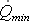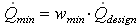# HOMER Pro 3.11Type: Intermediate Variable Units: m3/s Symbol:The minimum flow rate is the minimum allowable flow rate through the hydro turbine. HOMER assumes that the hydro turbine can operate only if the available stream flow is equal to or exceeds this minimum value. HOMER calculates the minimum flow rate of the hydro turbine using the following equation:where: wmin = the hydro turbine's minimum flow ratio [%]= the hydro turbine's design flow rate [m3/s]

HOMER uses this value to calculate the hydro turbine flow rate in each time step.